# Theoretical Mathematics

Theoretical mathematics is the study of abstract mathematical structures which form the basic framework for the rest of the mathematical sciences. In large part, theoretical mathematics is inspired by intellectual curiosity. Theoretical mathematics provides the tools for scientific discoveries in the future, often in unexpected ways.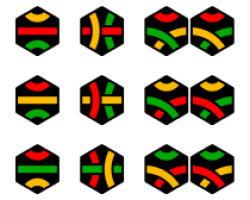## Discrete Mathematics

Algebra includes the study of groups, rings, fields, and vector spaces which are the basic frameworks from which to study many other areas of mathematics and science. Combinatorics is the study of counting the number of possibilities that meet a certain criteria. This subject closely relates to probability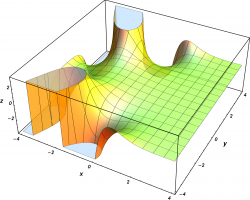## Analysis and Partial Differential Equations

Analysis includes operator theory and C*-algebras – the study of infinite dimensional systems of equations,  Fourier analysis – the study of infinite dimensional trigonometric systems, complex analysis – the study of functions of a complex variable, partial differential equations and dynamical systems – the equations which model how physical quantities change with time or space,  and research in theoretical and mathematical physics.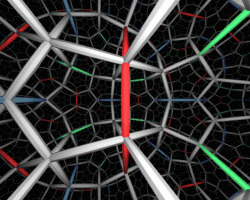## Geometry and Topology

Geometry and topology is the study of how objects in space bend or twist. It also involves categorizing objects based on properties such as the number of holes that are present or their symmetry.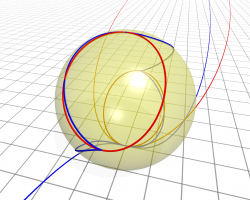## Number Theory

Number theory is the study of integers and fractions (rational numbers) – how they factor and how they arise as solutions to equations.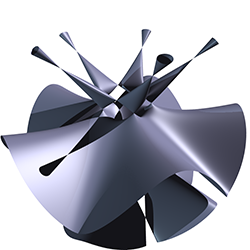## Algebra

Algebra is the study of algebraic structures such as groups, rings, and fields, and how they relate to other branches of mathematics including algebraic geometry, combinatorics, and representation theory.